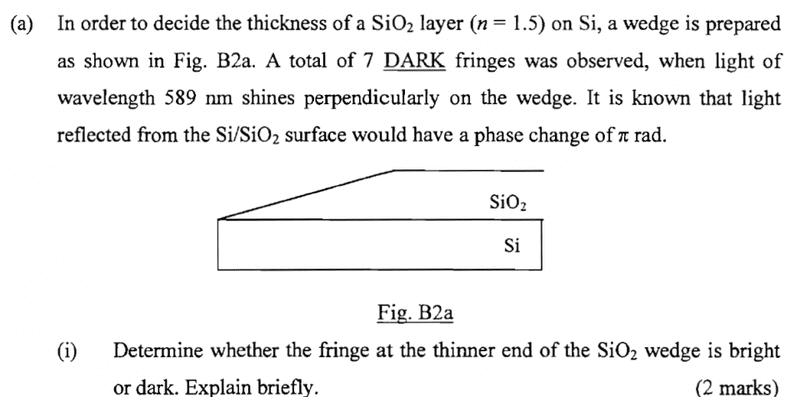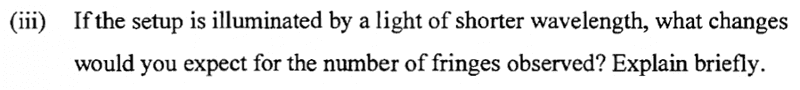# About thin film (max bright/dark)

## Homework Statement## The Attempt at a Solution

SiO2 as the thin film,when light entry SiO2 and Si then the reflected light has 180 phase change,
for dark fringes
2nt=(m+1/2)λ
for bright fringes
2nt=mλ
for dectect max of bright/dark,-1<=sinx<=1, then no idea to prove father[/B]

Simon Bridge
Homework Helper
Relate the thickness of the wedge to the wavelength of light ... notice there are seven fringes, so how many wavelengths of light thick is the widest part of the wedge?

Relate the thickness of the wedge to the wavelength of light ... notice there are seven fringes, so how many wavelengths of light thick is the widest part of the wedge?
could you possibly explain more? still not understand..

ehild
Homework Helper

## The Attempt at a Solution

SiO2 as the thin film,when light entry SiO2 and Si then the reflected light has 180 phase change,
for dark fringes
2nt=(m+1/2)λ
for bright fringes
2nt=mλ[/B]
Do you know what "t"means?
How thick is the oxide at the thinnest part?

Do you know what "t"means?
How thick is the oxide at the thinnest part?
t=the thick of SiO2

ehild
Homework Helper
t=the thick of SiO2
The SiO2 thickness changes, as it is a wedge. You see a bright strip where the thickness t matches the condition 2nt = mλ.
How thick is the SiO2 wedge at the thinnest part? Do you see it bright or dark?